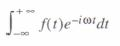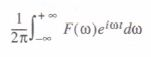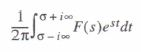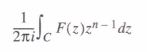This section describes how to perform the Fourier, Laplace, and z-transforms, and their inverses. The examples in this section demonstrate “live” transformations using symbolic keywords, but you may apply the Transforms commands from the Symbolics menu to expressions on a case by case basis if you prefer. Keep in mind that, unlike keyword-modified expressions, expressions modified by commands from the Symbolics menu do not update automatically, as described in the section ”Using the Symbolics menu”.

Figure 17-20 shows some examples of symbolic transforms in Mathcad. Note that the result may contain functions that are recognized by Mathcad’s symbolic processor but not by its numeric processor. An example is the function Dirac in the middle of Figure 17-20. You’ll find numerical definitions for this and other such functions at the end of this chapter and in the on-line Help.

When you perform a symbolic transform, Mathcad returns a function in a variable commonly used in the context of the transform. You can substitute a different variable for the one Mathcad returns by using the substitute keyword. See the section “Symbolic algebra” for information on substituting one variable for another.

Fourier and inverse Fourier transformations

To evaluate the Fourier transform of a function, use the keyword fourier:

• Enter the expression to be transformed.
• Press [Ctrl] [Shift 1 . (hold down the control and shift keys and type a period). Mathcad displays a placeholder to the left of the arrow, “→”.
• In the placeholder, type fourier followed by a comma and the transform variable.
• Press [Enter] to see the result.

Mathcad returns a function of co given by:wheref(t) is the expression to be transformed.

Mathcad returns a function in the variable cowhen you perform a Fourier transform since this is a commonly used variable name in this context. If the expression you are transforming already contain an to, Mathcad avoids ambiguity by returning a function of the variable ωω instead.

Another way to evaluate the Fourier transform of an expression is to enter the expression and click on the transform variable. Then choose Transferme⇒Fourier from the Symbolics menu.

To evaluate the inverse Fourier transform of a function, use the keyword invfourier:

• Enter the expression to be transformed.
• Press [Ctrl] [Shift 1 • (hold down the control and shift keys and type a period). Mathcad displays a placeholder to the left of the arrow, “→”.
• In the placeholder, type invfourier followed by a comma and the transform variable.
• Press [Enter] to see the result.

Mathcad returns a function of t given by:Laplace and inverse Laplace transformations

To evaluate the Laplace transform of a function, use the keyword laplace:

• Enter the expression to be transformed.
• Press [Ctrl] [Shift 1 • (hold down the control and shift keys and type a period).
Mathcad displays a placeholder to the left of the arrow, “~”.
• In the placeholder, type laplace followed by a comma and the transform variable.
• Press [Enter] to see the result.
Mathcad returns a function of 5 given by:\where f(t) is the expression to be transformed.
Mathcad returns a function in the variable 5 when you perform a Laplace transform since this is a commonly used variable name in this context. If the expression you are transforming already contains an 5, Mathcad avoids ambiguity by returning a function of the variable ss instead.

Another way to evaluate the Laplace transform of an expression is to enter the expression and click on the transform variable. Then choose Transformee-Laplace from the Symbolics menu.

To evaluate the inverse Laplace transform of a function, use the keyword invlaplace:

• Enter the expression to be transformed.
• Press [Ctrl] [Shift 1 • (hold down the control and shift keys and type a period).
Mathcad displays a placeholder to the left of the arrow, “~”.
• In the placeholder, type invlaplace followed by a comma and the transform variable.
• Press [Enter] to see the result.
Mathcad returns a function of t given by:where F(s) is the expression to be transformed and all singularities of F(s) are to the left of the line Re( s) = a. Mathcad returns a function in the variable t when you perform an inverse Laplace transform since this is a commonly used variable name in this context. If the expression you are transforming already contains a t, Mathcad avoids ambiguity by returning a function of the variable tt instead.

Another way to evaluate the inverse Laplace transform of an expression is to enter the expression and click on the transform variable. Then choose Transformeefnverse Laplace from the Symbolics menu

z and inverse z-transformations

To evaluate the z-transform of a function, use the keyword ztrans:

• Enter the expression to be transformed.
• Press [Ctrl] [Shift 1 . (hold down the control and shift keys and type a period). Mathcad displays a placeholder to the left of the arrow, “→”.
• In the placeholder, type ztrans followed by a comma and the transform variable.
• Press [Enter] to see the result.
Mathcad returns a function of z given by:where fin) is the expression to be transformed.

Mathcad returns a function in the variable z when you perform a z-transform since this is a commonly used variable name in this context. If the expression you are transforming already contains a z, Mathcad avoids ambiguity by returning a function of the variable zz instead.

Another way to evaluate the z-transform of an expression is to enter the expression and click on the transform variable. Then choose Transform⇒z- Transform from the Symbolics menu.

To evaluate the inverse z-transform of a function, use the keyword invztrans:

• Enter the expression to be transformed.
• Press [Ctrl] [Shift 1 • (hold down the control and shift keys and type a period).
Mathcad displays a placeholder to the left of the arrow, “→”.
• In the placeholder, type invztrans followed by a comma and the transform variable.
• Press [Enter] to see the result.
Mathcad returns a function of n given by a contour integral around the origin:where F(z) is the expression to be transformed and C is a contour enclosing all singularities of the integrand.  Mathcad returns a function in the variable n when you perform an inverse z-transform since this is a commonly used variable name in this context. If the expression you are transforming already contains an n, Mathcad avoids ambiguity by returning a function of the variable nn instead.

Another way to evaluate the inverse z-transform of an expression is to enter the expression and click on the transform variable. Then choose Transform⇒ lnverse z- Transform from the Symbolics menu.

Posted on November 23, 2015 in Symbolic Calculation